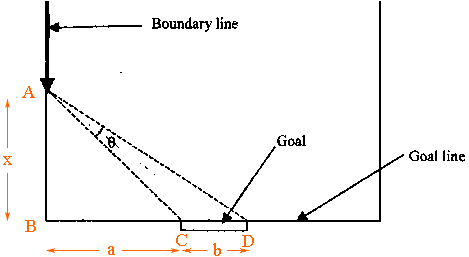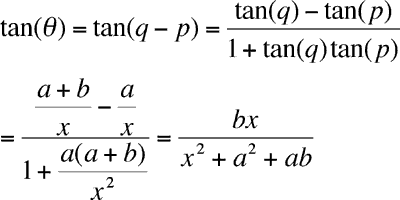Quandaries and Queries You are running down the boundary line dribbling the ball in soccer or hockey. Investigate where in your run the angle the goal mouth makes with your position is at a maximum. Yogendra Hi Yogendra, I added some labels to the diagram you sent.Let p be the measure of the angle CAB and q be the measure of the angle DAB, both in radians. Then using the diagram and some algebraic simplification I getTheta is a function of x so you can differentiate both sides with respect to x and find the value of x where the derivative of theta with respect to x is zero. Penny Go to Math Central